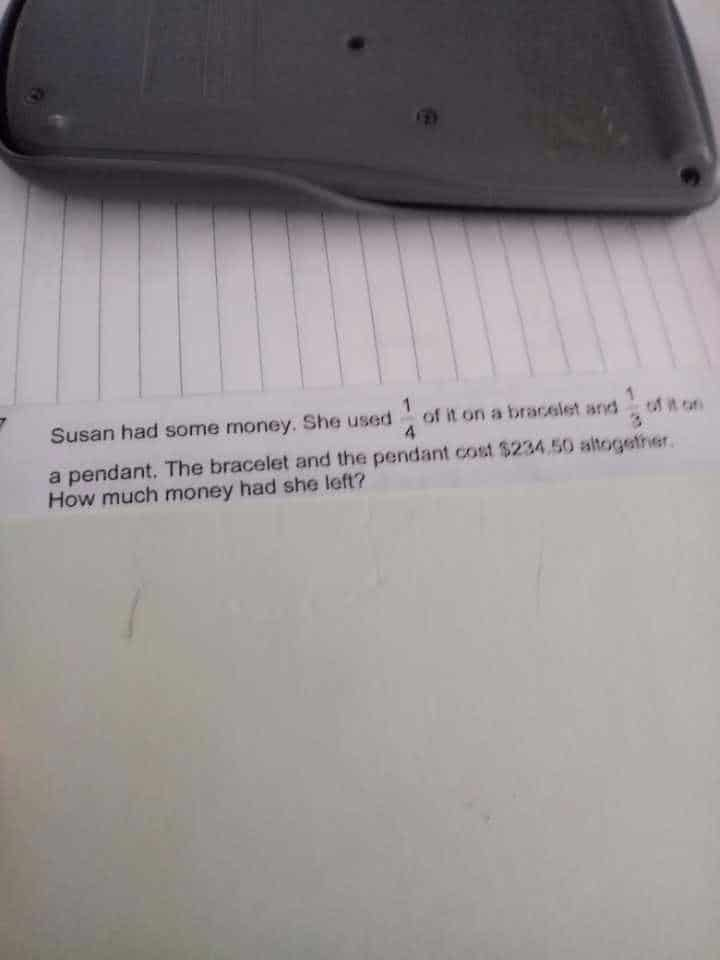QuestionThanks

This is a part whole question.

1.  Bracelet 1/4.  Pendant 1/3.

2.  LCM    1/4 = 3/12

1/3 = 4/12

 Bracelet Pendant Left Total Quantity 3u 4u 5u 12u

3u + 4u = 7u

7u = 234.50

1u = 234.5 ÷ 7 = 33.5

5u = 33.5 × 5 = \$167.5

😊

Note

When you see 2 fractions, find the LCM of the denominators .

When you see 2 fractions, and the secondone is a remainder of, then you use branching method.

When you see one fraction equal to another fraction, find the LCM of the numerators .

0 Replies 0 Likes